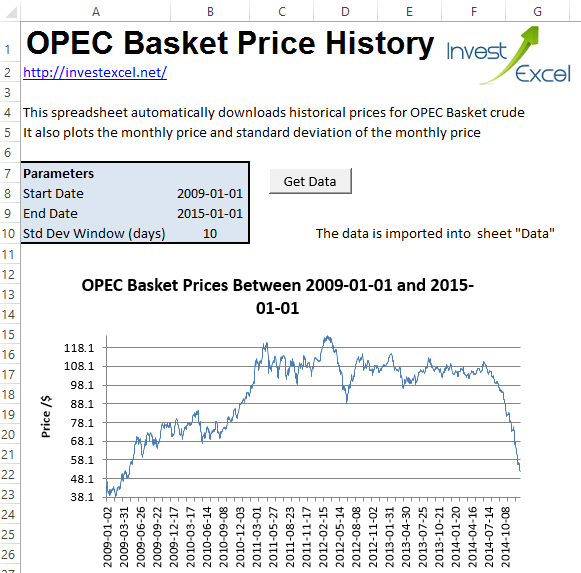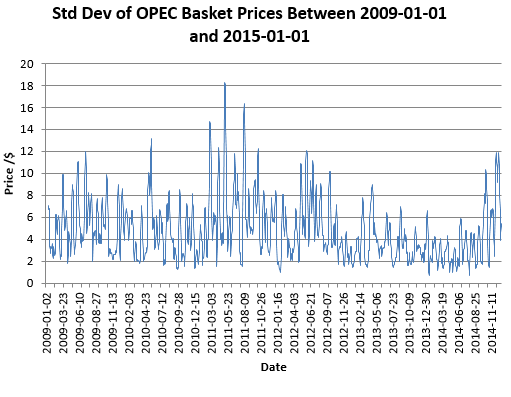# OPEC Basket Price History in Excel

You can analyze, slice and dice the crude oil prices – for example, calculating the correlation between oil and gold prices. You can do this with spreadsheet functions, or if you want far more flexibility then use VBA to automate your analysis.

The VBA is not hidden or locked away – just go to the Developer tab in Excel to view the code.

The code employs the Quandl API to import daily prices straight into a sheet. Just enter a couple of dates and hit a button; the spreadsheet does the hard work.Of course, you can now analyze the data; you might want to try, for example, calculating the correlation between equity and crude oil prices.

As an example of an analysis, the VBA in the spreadsheet calculates the rolling n-day standard deviation of the price history (set the value of n in the Parameter sheet). The formulas are automatically entered in the spreadsheet with VBA. Here’s the relevant part of the code.

```If SDWindow + 1 <= LastRow Then Sheets("Data").Range("C" & SDWindow + 1 & ":C" & LastRow).FormulaR1C1 = "=STDEV(R[-" & SDWindow - 1 & "]C[-1]:RC[-1])" Sheets("Data").Range("C" & SDWindow + 1 & ":C" & LastRow).NumberFormat = "0.00%" End If```

The VBA also generates two charts; one with the OPEC Basket price history, and another with the rolling standard deviation.The spreadsheet uses the Quandl API. This allows 50 anonymous API calls a day. If you refresh the spreadsheet more often than this, you’ll need to insert your Quandl author key into the url call in the VBA (if you need a hint, leave a comment).

Get OPEC Crude Oil Price History in Excel### 1 thought on “OPEC Basket Price History in Excel”

1. Really nice, Samir. Very fast. Knowledge is power!